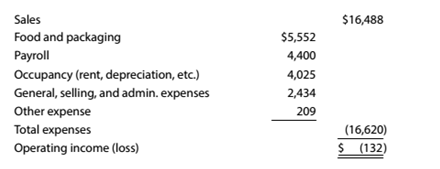Chapter 11, Problem 11.10E

Chapter
Section
Textbook Problem

Contribution margin and contribution margin ratio For a recent year. McDonald's (MCD) company-owned restaurants had the following sales and expenses (in millions):Assume that the variable costs consist of food and packaging, payroll, and 45% of the general, selling, and administrative expenses.a. What is McDonald's contribution margin? Round to the nearest million. b.What is McDonald's contribution margin ratio? Round to one decimal place. c. How much would operating income increase if same-store sales increased by $500 million for the coming year, with no change in the contribution margin ratio or fixed costs? d.What would have been the operating income or loss for the recent year if sales had been$500 million more? e.To achieve break even for the recent year, by how much would sales need to increase?

To determine

(a)

Concept introduction:

Contribution margin is the difference between the sales and variable cost. In other words, it is computed by subtracting thevariable cost from sales.

To compute:

The Contribution margin.

Explanation

Sale is $16,488 Variable Cost is$11,047.30 ($5,552+$4,400+($2,434×0 To determine (b) Concept introduction: Contribution margin is the difference between the sales and variable cost. In other words, it is computed by subtracting thevariable cost from sales. To compute: The Contribution Margin Ratio. To determine (c) Concept introduction: Operating income is earned by the business operations in the normal course of business. This income is computed by setting off all expenses from the sales revenue. The operating income increased in next year if sale increased by$500 Million.

To determine

(d)

Concept introduction:

Operating income is the one which is earned by the business operations in the normal course of business. This income is computed by setting off all expenses from the sales revenue.

The operating income for recent year if sale increased by \$500 Million.

To determine

(e)

Concept introduction:

The break-even point is the level of production at which the contribution earned recovers the fixed cost of the company, i.e., no profit no loss situation.

The sales that should be increased in current year so as to achieve the break-even.

Still sussing out bartleby?

Check out a sample textbook solution.

See a sample solution

The Solution to Your Study Problems

Bartleby provides explanations to thousands of textbook problems written by our experts, many with advanced degrees!

Get Started

Why would a firm ever offer a price on a product that is below its full cost?

Managerial Accounting: The Cornerstone of Business Decision-Making

SECURITY MARKET LINE You plan to invest in the Kish Hedge Fund, which has total capital of 500 million invested...

Fundamentals of Financial Management, Concise Edition (with Thomson ONE - Business School Edition, 1 term (6 months) Printed Access Card) (MindTap Course List)

How does a cost-efficient capital market help reduce the prices of goods and services?

Fundamentals of Financial Management, Concise Edition (MindTap Course List)